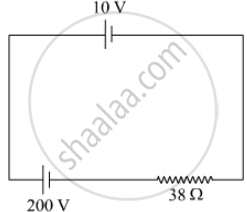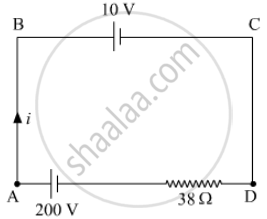Share

# A 10 V Cell of Negligible Internal Resistance is Connected in Parallel Across a Battery of Emf 200 V and Internal Resistance 38 ω as Shown in the Figure. Find the Value of Current in the Circuit. - CBSE (Science) Class 12 - Physics

#### Question

A 10 V cell of negligible internal resistance is connected in parallel across a battery of emf 200 V and internal resistance 38 Ω as shown in the figure. Find the value of current in the circuit.#### SolutionApplying KVL in the loop ABCDA

10 + 38i - 200 = 0

38i = 190

i = 190/38 = 5A

Is there an error in this question or solution?

#### Video TutorialsVIEW ALL 

Solution A 10 V Cell of Negligible Internal Resistance is Connected in Parallel Across a Battery of Emf 200 V and Internal Resistance 38 ω as Shown in the Figure. Find the Value of Current in the Circuit. Concept: Cells, Emf, Internal Resistance.
S